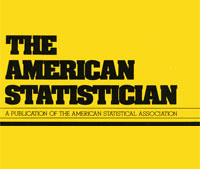## an improvable Rao–Blackwell improvement, inefficient maximum likelihood estimator, and unbiased generalized Bayes estimatorIn my quest (!) for examples of location problems with no UMVU estimator, I came across a neat paper by Tal Galili [of R Bloggers fame!] and Isaac Meilijson presenting somewhat paradoxical properties of classical estimators in the case of a Uniform U((1-k)θ,(1+k)θ) distribution when 0<k<1 is known. For this model, the minimal sufficient statistic is the pair made of the smallest and of the largest observations, L and U. Since this pair is not complete, the Rao-Blackwell theorem does not produce a single and hence optimal estimator. The best linear unbiased combination [in terms of its variance] of L and U is derived in this paper, although this does not produce the uniformly minimum variance unbiased estimator, which does not exist in this case. (And I do not understand the remark that

“Any unbiased estimator that is a function of the minimal sufficient statistic is its own Rao–Blackwell improvement.”

as this hints at an infinite sequence of improvement.) While the MLE is inefficient in this setting, the Pitman [best equivariant] estimator is both Bayes [against the scale Haar measure] and unbiased. While experimentally dominating the above linear combination. The authors also argue that, since “generalized Bayes rules need not be admissible”, there is no guarantee that the Pitman estimator is admissible (under squared error loss). But given that this is a uni-dimensional scale estimation problem I doubt very much there is a Stein effect occurring in this case.

This site uses Akismet to reduce spam. Learn how your comment data is processed.